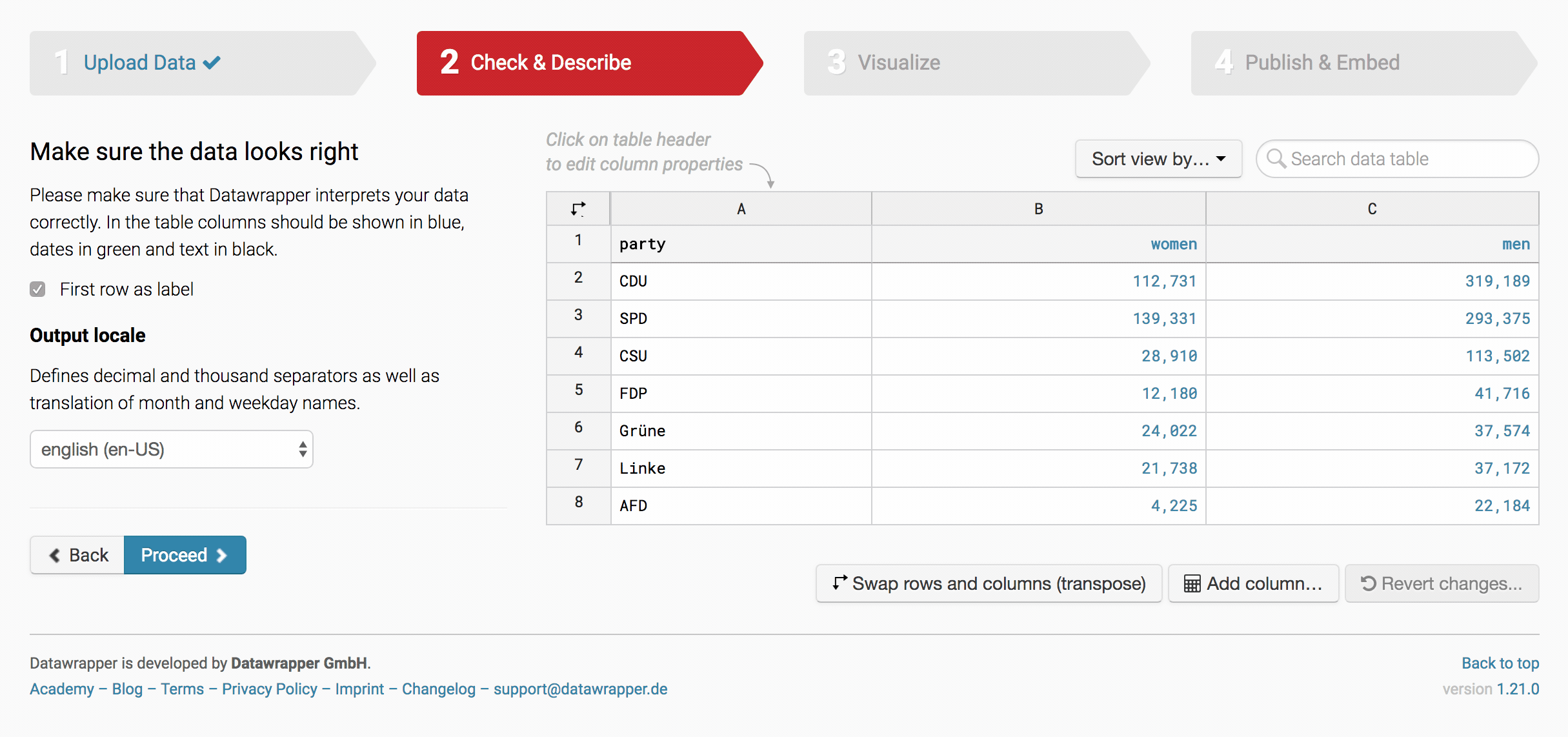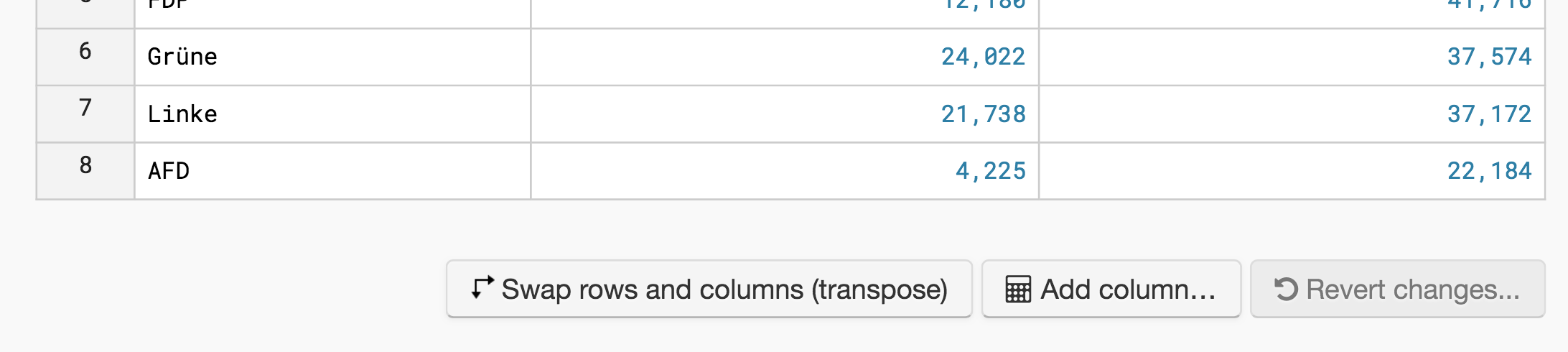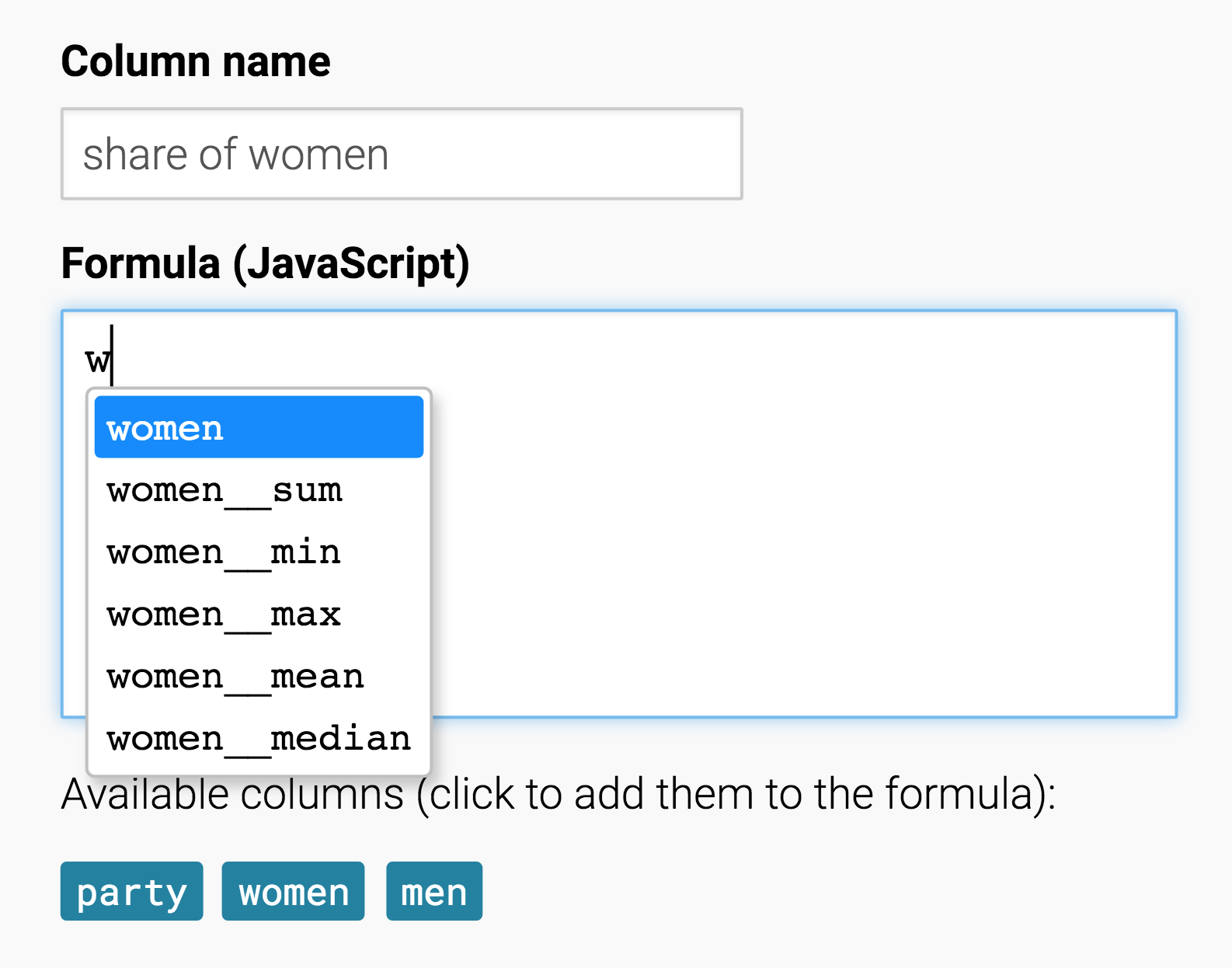# How to add new columns from existing dataYou've uploaded your data, but noticed that a calculation in your data is necessary? Adding two numbers, dividing a number by another number, etc. – you can do these calculations directly in Datawrapper. In step 2: Check & Describe, you can add a column based on values of other columns, similar to how you add columns in Excel. This posts explains how to use the feature.

### Example: Calculating the gender ratio for German parties

To begin with, let’s take a look at a simple dataset. We have the number of members of German parties by gender ( columns), for each of the seven parties (rows). Now how about adding a new column that contains the percentage of women for each party?

Click the button labeled “Add column…” below the table:Next, you can enter the column name, for instance, “share of women“. This will be the name the column appears as in the data table and in column selector drop-downs in the chart editor. You can use any character, but you cannot use a column name that already exists in the dataset.

Finally, you can enter the formula. You can refer to the values in other columns via their column name. Start by typing the first character of the column you want to address and then hit the Tab key to auto-complete. In case there are multiple columns starting with that letter you can either enter more characters or select the column from a little popup window.

This popup-window will also show you some Javascript options that we will explain at the end of this article (but that we won't use for our example; so you can ignore them for now).While you are typing the formula, a little display in the sidebar will show you how the first five computed values do look like. To compute the gender ratio for this example, we type the formula women / (men + women)*100.:That's it! You can always check the output of your calculations directly in the table. If you still want to edit the column format (e.g. adding suffixes or prefixes like percentage signs, or deciding how many decimal places should be displatyed), click on "Edit column format" at the bottom. There, you can also delete your calculated column:You can also add more than one column. Simply click the "Add column" button again.

### List of supported functions and variables

You can use access basic mathematic functions such as  round and column aggregates (sum, median etc). Here's a list of functions you can use in your formula:

 round( value, [precision] ) Round a number min( value1, value2 ) Returns the smallest of two numbers max( value1, value2 ) Returns the largest of two numbers median( values ) Returns the median value of an array of numbers mean( values ) Returns the arithmetic mean value of an array of numbers sum( values ) Returns the sum of an array of numbers

On top of that you can append the following suffixes to any numeric column name to get aggregated values from entire columns:

 column__sum Sum of all values in a column column__min Smallest value of column column__max Largest value of column column__mean Arithmetic mean of column column__median Median value of column __row The row number, starting from zero